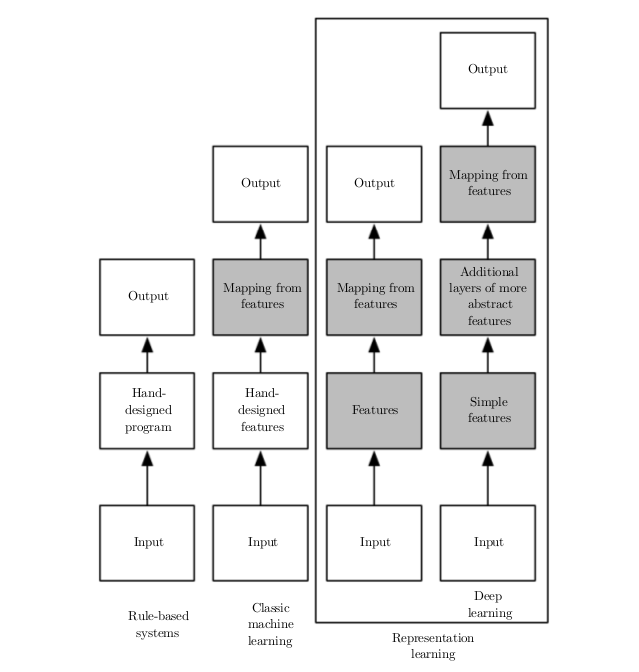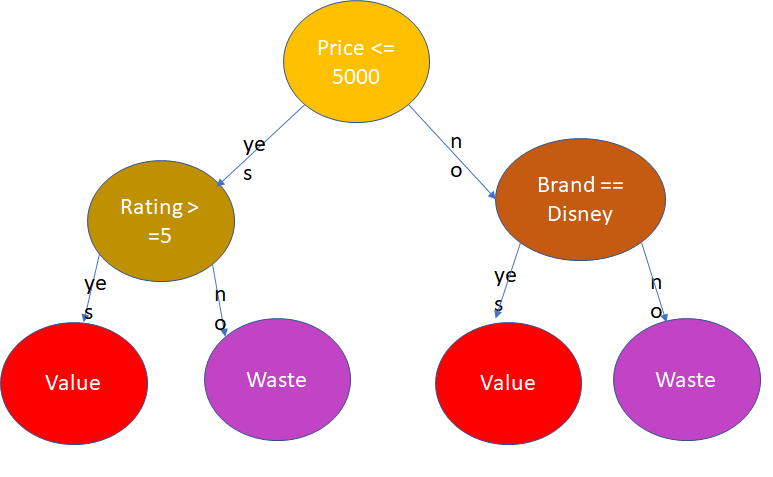• ABV-Indian Institute of Information Technology And Management, Gwalior

# Machine Learning : What, Why and How?

Nitish Bhardwaj

* * *

# Machine Learning : What, Why and How?

Artificial Intelligence, Machine Learning and Deep Learning are the terms you have been reading in almost every blog these days. I am going to walk you through the basic terminology of Machine Learning (ML).

What : Machine Learning (ML) is defined as “Program machines to learn and improve with experience”. So, you can think of ML as a small kid, who has not explored the world and you want to teach different things by showing him different examples. You also give feedback by asking questions. And over the time, the kid improves skills with experience. Now, you take the skilled kid and ask question and s(he) answers almost question perfectly.

So, let’s rephrase the above example with ML terminology : showing him different examples (training data), feedback by asking questions(training and validation loss and accuracy calculation), skills improvement (training) and skilled kid (trained model).

Why : Let’s dive more into ML with the same example, let’s now teach the kid to get better understanding of toys. There are multiple problems like Estimation of the price of the toy, Evaluation of worth of the toy or Selecting the best toy based on preferences. These problems can be restated as ML problems as Prediction, Classification and Recommendation respectively. So, ML is going to give a better understanding and solvers for many problems.

How : Machine Learning process works in the way we discussed about teaching a small kid to learn and improve with experience from the given examples. There are mainly 3 components : Data-Training-Testing. The complete process differs based on type-of-data, type-of-problem, process-of-learning and expected-output. The below diagram gives a basic flow of Learning process which has evolved over time.Flowcharts showing how the diﬀerent parts of an AI system relate to each other within diﬀerent AI disciplines.Shaded boxes indicate components that are able to learn from data. Reference :http://www.deeplearningbook.org/contents/intro.html

Let’s try to solve the problem of “Evaluation of worth of the toy”. There are no fixed rules, but I will define the process in 7 steps as follows :

1. Defining the Problem Statement : For example, let’s say given properties of some 10 toys, create a ML model to predict whether the toy is “value for money” or “waste of money”. Redefining ML problem as Classification : classes (two-classes or outcomes, viz., “value” and “waste”) and data (properties/features of toys)
2. Tagging Data : Defining features and outcomes, for given example, properties of toys like (price, rating, durability, brand_name, weight, color, etc….). Let’s define features like (price, rating, brand) as x and tag the class/outcome as y.
3. Selection of Training Algorithm : Most complex step, requires more understanding of problems and data, or just look for some standard library / framework / package for classification problem. Match the expected formats x and y for that library and use it. I will talk more about it the next blog.
4. Training Process: For the given x and y for the examples data and the selected algorithm, run the training and save the trained model. Trained model is a mathematical function which will return y for the input x. In this process, the algorithm tries to create this function which can justify all the outcomes(y) for every example inputs(x). The difference between the actual outcome and and the outcome predicted by partially trained model is called loss. The objective of training is to minimize the training loss.For example, the diagram below show a decision tree used for classification.Example of Decision Tree Algorithm for our given use-case

5. Validation of the trained model: This is an evaluation process, it is used to check validity of the trained model that how the model is going to perform when we will use it for classification for new examples which are not part of training data. This process can also be used while training (called cross-validation).

6. Re-training process : Based on the results of evaluation, we might need to train the model with different algorithm or we need to tweak around some parameters used for this algorithm (called Hyperparameter Tuning). It requires understanding of algorithm. After few-iteration from points 4–6, we get a final trained model.

7. Testing : The goal is for given properties of a new toy, predict whether the toy is “value for money” or “waste of money”. Extract features (x) of the new toy, use the trained model, and it will result y (“value” or “waste”) .

So, just start exploring the Machine Learning example, without any prerequisites. Please check the below link to see the working solution of given problem in less than 20 lines of Python code.

Thanks for reading the blog, feel free to drop suggestion or corrections.!!

References :

https://developers.google.com/machine-learning/crash-course/

https://www.youtube.com/watch?v=nKW8Ndu7Mjw